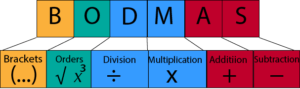Do you vaguely remember hearing the acronym BODMAS from school days?  It is also known as BOMDAS and in the US PEMDAS. It sets out the order of Mathematical Operations. What you may ask are Mathematical Operations? The simplest mathematical operations involve the operators    –   x   ÷   ie plus, minus, multiply and divide. These operators you are familiar with for doing simple arithmetic.

With a simple sum that only has two numbers and one single operator, it is easy to calculate the answer. Sometimes however there may be several numbers and different operations. So what do you do if in one sum you have to multiply, add, subtract and divide? As to be expected in Mathematics there are simple rules to follow to help you work out the order in which to do the calculations.

Brackets (any part contained in brackets comes first)

Order (operations containing powers or indices such as squaring or square rooting)

Division and Multiplication

Addition and Subtraction

## 7 –  1  x  0 +  3  ÷ 3  = ?

With this in mind in 2012 Dr Peter Price, the co-founder of the Classroom Professor website, posted a mathematical problem on his facebook page. Consequently seventy thousand people saw the post with 6,000 leaving answers and comments. However after 2 weeks, the results were studied and only 26% had the correct answer. The answer is 8. Most people who posted an answer would have been fairly confident of their answer so as not to look foolish on a web site. So this appears to demonstrate that the large majority of the population (probably much more than 74%) do not understand the concept of the order of operations.

Fortunately this is where BODMAS comes in.

In the sum 7 x 3 + 5 there are three numbers and two operators a multiplication (x) and an addition (+).

If you multiply seven by three and add five the answer is 26.

If you multiply seven x (three plus five), the answer is 56. So which is correct?

On the positive side there is a defined order summed up by BODMAS.* In the USA it is normally called PEMDAS (Parenthesis, Exponent, Multiply, Divide, Add, Subtract).

## Applying the order of operations

### Step 1:  Brackets

Firstly the highest level order is defined by anything in brackets. Consequently these are always calculated first. If there is more than one set of brackets start at the innermost set and work outwards and you should be left with one number.

### Step 2: Order or Index

These relate to squaring or square rooting and should be done next.

### Steps 3 and 4:  Divide and Multiply

Thirdly division and multiplication are next As they have equal weight and and if there is more than one operators the calculation is carried out right to left.

Therefore 18 ÷ 6 x 4 ÷ 8 is worked from left to right. Eighteen divided by 6 is three and three times four is twelve, divided by 8 is 1.5.

### Steps 5 and 6: Add and Subtract

Again these carry equal weight so the sum is carried out right to left.

## 5 – 3 x 0 + 30 ÷ 5 = ?

The answer is at the end of the blog.

Our Letters and Numbers app uses BODMAS. You are given a target number and asked to arrive at the answer by using a set of numbers and operators and brackets. Below is an example.How close can you get to 676 using the numbers and operators shown.You can get to 672 by doing the following sum (75+25)x(9×8).

There was one problem where the first 200 people could not even get close. The number was 813 and the numbers were 100, 75, 50, 25, 3 and 1.You can download the app for free and practice using BODMAS in free mode.

Click on the link below.

https://itunes.apple.com/us/app/countdown-letters-and-numbers/id1219218384?ls=1&mt=8

The answer to the second sum is 11.

## 3 thoughts on “How does Bodmas make a difference?”

1. Excellent rundown of the sequences we should use to get the correct answer and the opportunity to practise.

2. Good to revise things we learnt so long ago!